# The QLIM Procedure

### Heteroscedasticity and Box-Cox Transformation

Subsections:

#### Heteroscedasticity

If the variance of regression disturbance, (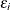), is heteroscedastic, the variance can be specified as a function of variables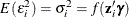The following table shows various functional forms of heteroscedasticity and the corresponding options to request each model.

 No. Model Options 1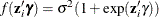LINK=EXP (default) 2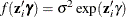LINK=EXP NOCONST 3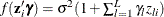LINK=LINEAR 4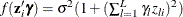LINK=LINEAR SQUARE

For discrete choice models,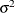is normalized (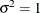) since this parameter is not identified. Note that in models 3 and 5, it may be possible that variances of some observations are negative. Although the QLIM procedure assigns a large penalty to move the optimization away from such region, it is possible that the optimization cannot improve the objective function value and gets locked in the region. Signs of such outcome include extremely small likelihood values or missing standard errors in the estimates. In models 2 and 6, variances are guaranteed to be greater or equal to zero, but it may be possible that variances of some observations are very close to zero. In these scenarios, standard errors may be missing. Models 1 and 4 do not have such problems. Variances in these models are always positive and never close to zero.

The heteroscedastic regression model is estimated using the following log-likelihood function: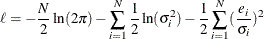where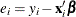.

#### Box-Cox Modeling

The Box-Cox transformation on x is defined as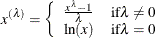The Box-Cox regression model with heteroscedasticity is written as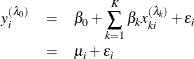where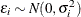and transformed variables must be positive. In practice, too many transformation parameters cause numerical problems in model fitting. It is common to have the same Box-Cox transformation performed on all the variables — that is,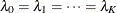. It is required for the magnitude of transformed variables to be in the tolerable range if the corresponding transformation parameters are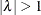.

The log-likelihood function of the Box-Cox regression model is written as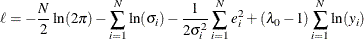where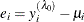.

When the dependent variable is discrete, censored, or truncated, the Box-Cox transformation can be applied only to explanatory variables.